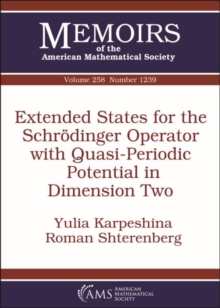Extended States for the Schrodinger Operator with Quasi-Periodic Potential in Dimension Two Paperback / softback

Part of the Memoirs of the American Mathematical Society series

Description

The authors consider a Schrodinger operator $H=-\Delta +V(\vec x)$ in dimension two with a quasi-periodic potential $V(\vec x)$.

They prove that the absolutely continuous spectrum of $H$ contains a semiaxis and there is a family of generalized eigenfunctions at every point of this semiaxis with the following properties.

First, the eigenfunctions are close to plane waves $e^i\langle \vec \varkappa ,\vec x\rangle$ in the high energy region.

Second, the isoenergetic curves in the space of momenta $\vec \varkappa$ corresponding to these eigenfunctions have the form of slightly distorted circles with holes (Cantor type structure).

A new method of multiscale analysis in the momentum space is developed to prove these results. The result is based on a previous paper on the quasiperiodic polyharmonic operator $(-\Delta )^l+V(\vec x)$, $l>1$.

Here the authors address technical complications arising in the case $l=1$.

However, this text is self-contained and can be read without familiarity with the previous paper.

Information

• Format: Paperback / softback
• Pages: 139 pages
• Publisher: American Mathematical Society
• Publication Date:
• Category: Differential calculus & equations
• ISBN: 9781470435431

£79.95

£79.25

on all orders

Pick up orders

from local bookshops

£79.95

£79.25

£79.95

£79.25

£79.95

£79.25

£79.95

£79.25